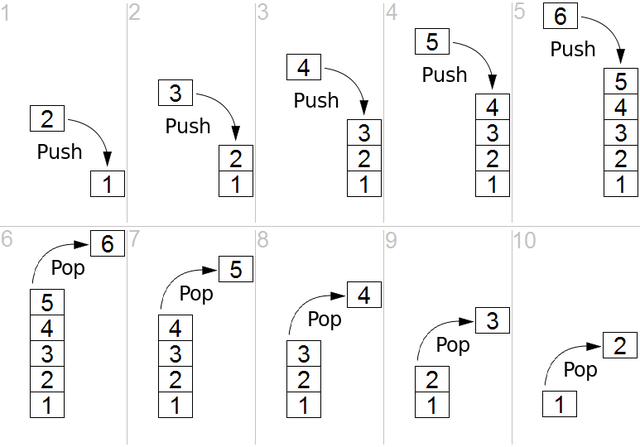# Stack Class in Java Explained with Examples

Jan 26, 2020 · 5 mins readStack is a data structure that holds a collection of elements (e.g. integers) and supports two basic operations on them: push and pop:

• push: add an element to the collection
• pop: remove the most recently added element

Because of the way elements are removed from it, stack is also known as last in, first out (LIFO) data structure.

Java collections framework comes with a built-in implementation of the Stack data structure in the form of the `java.util.Stack` class. In addition to the basic push and pop operations, the `Stack` class also supports other methods such as peek to look at the top item of the stack without removing it, a method for testing whether the stack is empty and a method for searching the stack.

Let’s take a look at an example. In this example, we’ll create a stack to hold integer objects and illustrate the use of stack operations: `push()` and `pop()`. We’ll add 6 items to the stack (numbers 1 through 6) and pop them from the stack until it is empty as illustrated in the figure below. This code was tested on Java 8.``````import java.util.Stack;

public class StackExample {

public static void main(String[] args) {
// Create a new stack
Stack<Integer> stack = new Stack<>();

// Push 6 items
stack.push(1);
stack.push(2);
stack.push(3);
stack.push(4);
stack.push(5);
stack.push(6);

// Pop items from the stack until its empty
while (!stack.empty()) {
System.out.println(stack.pop());
}
}
}
``````

This program will print:

``````6
5
4
3
2
1
``````

In the next example, let’s take a look at the following methods of the `Stack` class:

• peek(): Returns the item at the top of this stack without removing it from the stack.
• empty​(): Returns `true` if the stack is empty. `false` otherwise.
• search(Object o): Returns position of the item (passed as argument) on stack measured in terms of its distance from the top. This method counts from 1 instead of 0 (weird) so if the item is found at the top of the stack, it’ll have position 1. If the item isn’t found, it will return -1.
``````import java.util.Stack;

public class StackExample2 {
public static void main(String[] args) {

// Create a new stack
Stack<String> stack = new Stack<>();

System.out.println("new stack is empty: " + stack.empty());

// Push some items to it
stack.push("out");
stack.push("is");
stack.push("13");
stack.push("java");

// peek at the top item
System.out.println("peek(): " + stack.peek());

// search for a valid item
System.out.println("search for 'java': "+ stack.search("java"));

// pop all elements: can you GUESS the output?
while (!stack.empty()) {
System.out.print(stack.pop() + " ");
}
}
}
``````

The code above will print:

``````new stack is empty: true
peek(): java
searching for 'java': 1
java 13 is out
``````

Notes:

• A stack is used for depth-first search implementations.
• Stacks are also used in computing architectures for memory layout of processes. Each process (or thread) gets a reserved region of memory that acts like a stack. When a function is called, it is added to the top of the stack along with its data. When a function exits, it is removed from the stack.
• In practice, if you need support for LIFO operations, an implementation of the Deque interface should be preferred over the Stack class. For example, you can use ArrayDeque class.
• If you’re interested in a barebones implementation of the stack data structure, here’s a good implementation using linked list.

## Method Summary

Method Description Example
boolean empty() Tests if this stack is empty. if (stack.empty())
System.out.print("empty stack");

E peek() Looks at the object at the top of stack without removing it. assert (stack.peek() == 2 * a);
E pop() Removes the object at the top of this stack and returns it. Integer num = stack.pop();
E push(E item) Pushes an item onto the top of this stack. stack.push("Ace of Spades");
int search(Object o) Return 1-based position where object o is on this stack. Integer pos = stack.search(element);

if(pos == -1)